Quantity: 0

Total: 0,00

0

# Regular square pyramid

### Regular square pyramid

A regular square pyramid is a right pyramid with a square base and four triangular faces.

Mathematics

Keywords

mathematics, geometry, solid geometry, solids, grouping of solids, pyramid, surface, volume, definition, motherboard, lateral surface, face, height, formula, right pyramid, tetrahedron, regular solids, oblique pyramid, vertices, faces, edges

Related items

### Scenes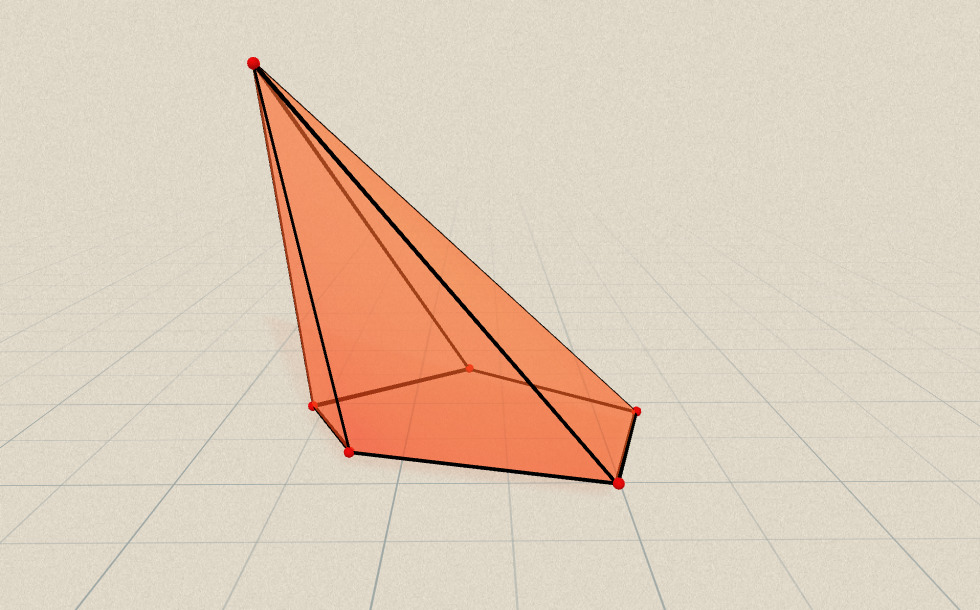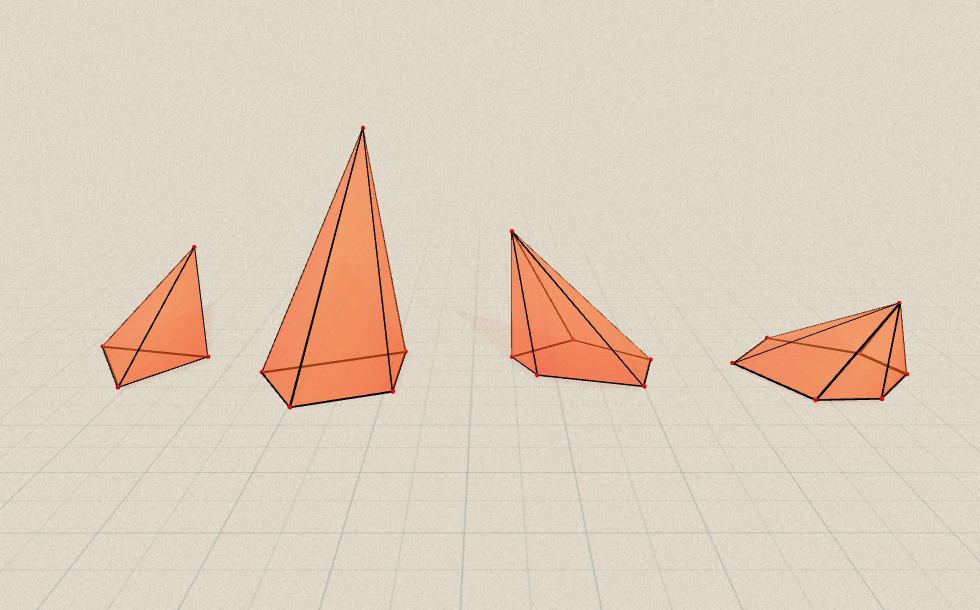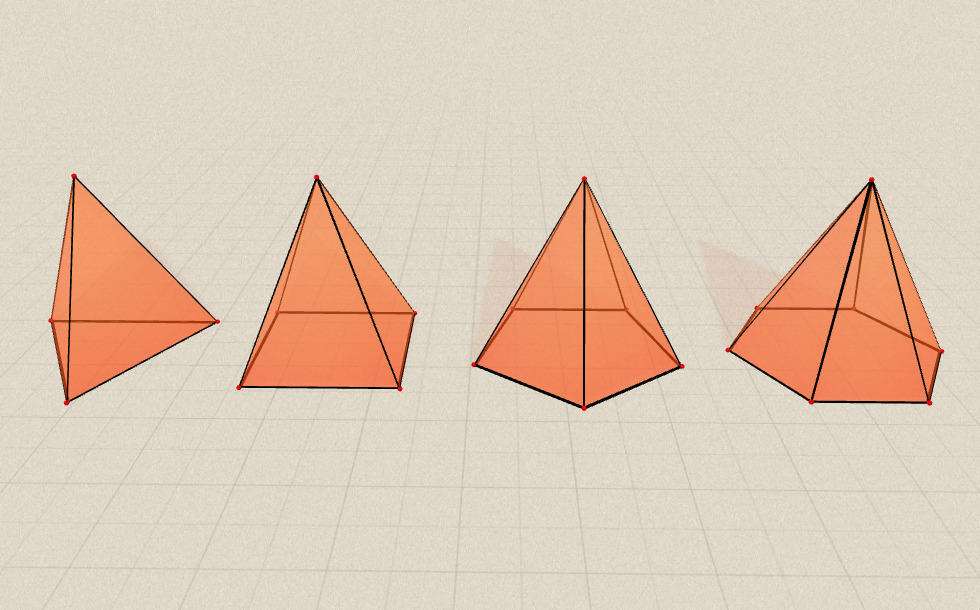The base of a regular pyramid is a regular polygon and its lateral edges are equal in length. (Therefore the lateral faces are are congruent isosceles triangles.) In regular pyramids the base point of the height coincides with the center of the base.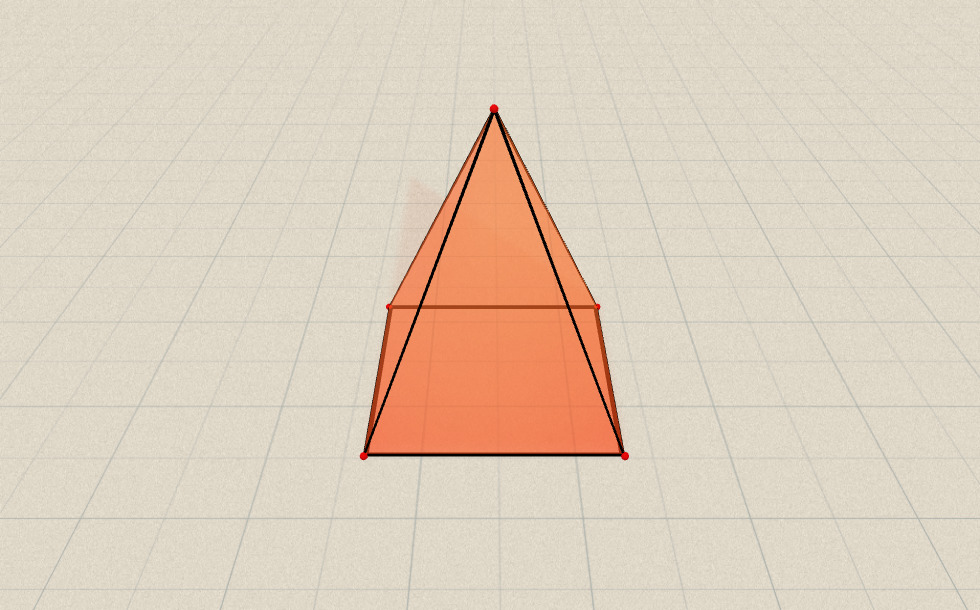The base of a regular square pyramid is a regular quadrilateral, i.e. a square. Its base edges (a) are equal in length and so are the lateral edges (b). Therefore, the lateral faces are isosceles triangles. In regular pyramids the base point of the height coincides with the center of the square base (O).

The lateral faces of the pyramid form the lateral surface, which, in the case of a regular square pyramid, comprises four congruent isosceles triangles. The area of such a triangle is half the product of its base length and its height. (In other words, it is half the product of the pyramid’s base edge (a) and the slant height (l).) The area of the base is the square (a²) of the length of base edges (a). The surface area of the pyramid is the sum of the base area and the lateral surface area (the sum of the areas of the lateral faces).

To calculate the volume of the pyramid, we can start with the volume of a prism with the same base and height as those of the pyramid. The volume of the prism is the product of its base area (A) and its height (h). The volume of the pyramid is one third the volume of the prism. In other words, the volume of the pyramid is one third the product of its base area and its height.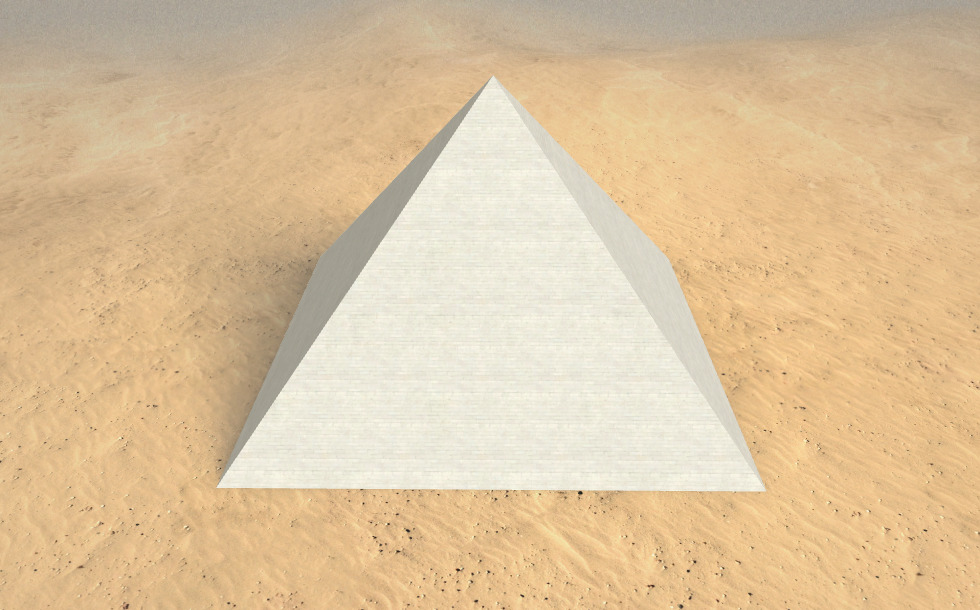### Related items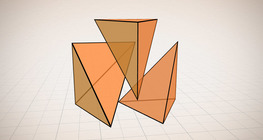#### Volume of a tetrahedron

To calculate the volume of a tetrahedron we start by calculating the volume of a prism.#### Cube sections (exercise)

Examining solids formed by the intersection of a cube and a plane.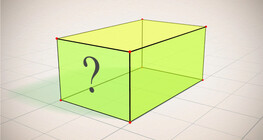#### Cuboid (exercises)

Edges, diagonals and faces of a cuboid can be identified by its vertices.#### Euler's polyhedron formula

The theorem formulated by Leonhard Euler describes one of the basic properties of convex polyhedra.#### Grouping of solids 1

This animation demonstrates various groups of solids through examples.#### Grouping of solids 2

This animation demonstrates various groups of solids through examples.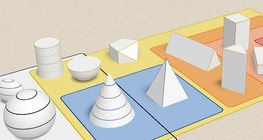#### Grouping of solids 3

This animation demonstrates various groups of solids through examples.#### Grouping of solids 4

This animation demonstrates various groups of solids through examples.#### Perimeter, area, surface area and volume

This animation presents the formulas to calculate the perimeter and area of shapes as well as the surface area and volume of solids.#### Platonic solids

This animation demonstrates the five regular three-dimensional (or Platonic) solids, the best known of which is the cube.#### Ratio of volumes of similar solids

This 3D scene explains the correlation between the ratio of similarity and the ratio of volume of geometric solids.#### Solids of revolution

Rotating a geometric shape around a line within its geometric plane as an axis results in a solid of revolution.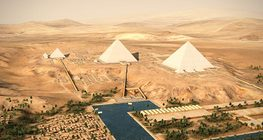#### Egyptian Pyramids (Giza, 26th century B.C.)

The Giza Necropolis is the only one of the Ancient wonders still intact.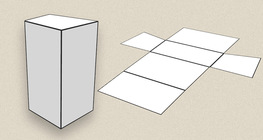#### Prisms

This animation demonstrates several types of prisms, from general to regular.